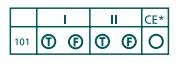# SAT Chemistry Subject Test Question Types

## SAT Chemistry Subject Test Question Types

The SAT Chemistry Subject Test was formulated and structured by College Board to assess the necessary skills and potentials of a student aspiring to study science or engineering at college level. The test is highly subject-oriented helping applicants in showcasing their interests and strengths in terms of various concepts related to Chemistry.

Apart from just focussing on the theory-related preparations, one must understand the different types of questions that appear on a usual CHEMISTRY SAT SUBJECT TEST. The key to attaining a high score on the test is by consistent practice of those question types and formats frequently appearing on the test. Majority of questions asked on the test are based upon application and synthesis of knowledge and a very small proportion are direct-theory related. The 3 main types of questions appearing on SAT CHEMISTRY test are as follows.

Type I: Five-choice Completion

These are the multiple choice questions which stand-alone. The student has to pick out the right answer choice. Sometimes, there will be a list of three statements in roman numerals and the test-taker has to pick out the correct option. Here’s an example to understand these formats in detail:

*1. An atom of beryllium consists of 4 protons, 5 neutrons, and 4 electrons. The mass number of this atom is

• 13
• 9
• 8
• 5

The aim is to study what constitutes the mass number.

Mass Number = Sum of the total number of Protons and Neutrons in the nucleus of an atom.

Hence, the correct answer choice is (B).

Mass Number= Protons + Neutrons = 4 + 5= 9

*2. The placement of the halogen family in the Periodic Table explains which of the following statements?

1. The most active non-metallic element in the periodic table is fluorine.
2. The normal physical state of the halogens goes from a solid to a gaseous state as you go down the family.
• The halogen elements become ions by filling the outermost d orbital.
• I only
• II only
• I and II
• II and III
• I and III

The aim is to analyse the 3 different statements separately and then pick out the correct statement or statements. Finally, mark the correct option representing the chosen true statement/statements.

Let’s look at the explanation to the 3 statements.

Statement I deals with the reactivity of fluorine. Since, it is smallest element as well as the most electronegative non metal, statement I is true.

Statement II is wrong as the physical state of halogens goes from gas to liquid to solid down the group.

Statement III is wrong as the halogens become ions by completing the outer p orbital.

So, the only correct statement is I. Hence, the right answer choice is (A).

Type II: Classification

There will be one list of options relevant to a set of questions that follows these choices. A given option can be used more than once or it may not be an answer to any of the questions that follow. So, do not panic if any of the choices does not appear as an answer to the questions. Take a look at an example:

*1. For the questions 1-3, complete the sentence with the appropriate choice.

• the free energy is always negative.
• the free energy is negative at lower temperatures.
• the free energy is negative at high temperature.
• the free energy is never negative.
• the system is at equilibrium, and there is not reaction.
1. When enthalpy is negative and entropy is positive,
2. When enthalpy is positive and entropy is positive,
3. When enthalpy is negative and entropy is negative,

Most of these questions are quite straight-forward.

The correct answers to the above questions are:

1. When enthalpy is negative and entropy is positive, the fee energy is always (A).
2. When enthalpy is positive and entropy is positive, the free energy is negative at high temperatures (C).
3. When enthalpy is negative and entropy is negative, the free energy is negative at lower temperatures (B).

Type III: Relationship Analysis

The question comprises of two statements connected to each other by ‘BECAUSE’. These question types are based on fundamental chemical facts on which the processes or properties depend. To make it clear, take a look at the following example:

I

*1.NACL is an example of ionic bonding

BECAUSE

II

Na and Cl have the same electronegativity.

Statement I is true as the electronegativity difference between sodium and chlorine is 1.7.

Statement II is false as they do not have the same electronegativity as Sodium is a metal and chlorine is a non-metal.So, your response should be shaded according to the correct answer. For statement I, shade ‘T’ and statement II, shade ‘F’. Since, second statement is false, leave the last column labelled as ‘CE’ which stands for Correct Explanation. This has to be taken into consideration if and only if the given two statements are true and check for the fact that statement I is true because of statement II.

Students need to practice on these 3 types of question formats to secure a high score in the Subject Test.

At Option SAT Dubai, the trainers provide personalised attention to the students in understanding the theory-related concepts and how effectively one can master these tricky question-types.

Good Luck to all Aspirants!!!

*Sourced from Barron’s Sat Subject Test Chemistry 12th Edition

Recommended Posts